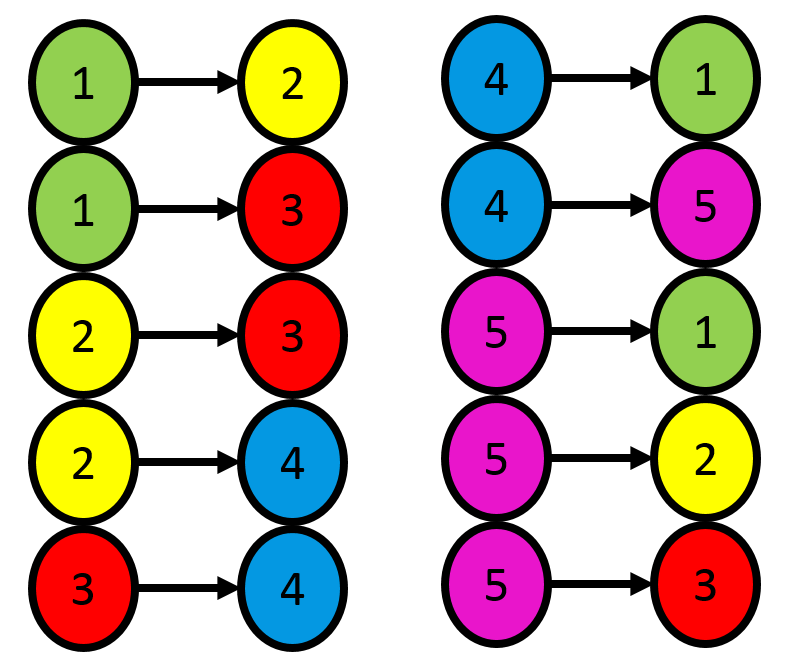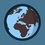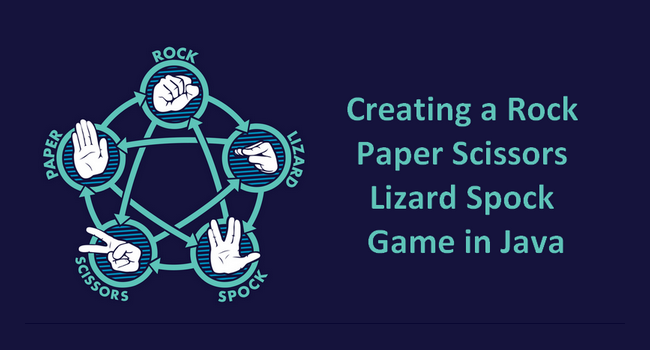# Extended "stone paper scissors" gameIn the game "stone paper scissors" we have 3 options. Now extend the game. There are 5 opportunities: $$1,2,3,4,5$$. The rules: $$1\rightarrow 1,2; 2\rightarrow 3,4; 3\rightarrow 4; 4\rightarrow 1,5; 5\rightarrow 1,2,3$$.($$a\rightarrow b$$ means that a hit b). The chance to win with $$1,2,3,4,5$$ are $$a,b,c,d,e$$ in this order. What are a,b,c,d,e?

P.S. I think we choose an option with a chance equal to the chance of winning with it. For example we choose the first option with $a$ chance.Note by Páll Márton (no activity)
4 months ago

This discussion board is a place to discuss our Daily Challenges and the math and science related to those challenges. Explanations are more than just a solution — they should explain the steps and thinking strategies that you used to obtain the solution. Comments should further the discussion of math and science.

When posting on Brilliant:

• Use the emojis to react to an explanation, whether you're congratulating a job well done , or just really confused .
• Ask specific questions about the challenge or the steps in somebody's explanation. Well-posed questions can add a lot to the discussion, but posting "I don't understand!" doesn't help anyone.
• Try to contribute something new to the discussion, whether it is an extension, generalization or other idea related to the challenge.

MarkdownAppears as
*italics* or _italics_ italics
**bold** or __bold__ bold
- bulleted- list
• bulleted
• list
1. numbered2. list
1. numbered
2. list
Note: you must add a full line of space before and after lists for them to show up correctly
paragraph 1paragraph 2

paragraph 1

paragraph 2

[example link](https://brilliant.org)example link
> This is a quote
This is a quote
    # I indented these lines
# 4 spaces, and now they show
# up as a code block.

print "hello world"
# I indented these lines
# 4 spaces, and now they show
# up as a code block.

print "hello world"
MathAppears as
Remember to wrap math in $$ ... $$ or $ ... $ to ensure proper formatting.
2 \times 3 $2 \times 3$
2^{34} $2^{34}$
a_{i-1} $a_{i-1}$
\frac{2}{3} $\frac{2}{3}$
\sqrt{2} $\sqrt{2}$
\sum_{i=1}^3 $\sum_{i=1}^3$
\sin \theta $\sin \theta$
\boxed{123} $\boxed{123}$

Sort by:

\left\{\begin{matrix}\begin{aligned} a&=\left(b+c\right)+a\left(b+c\right)+a^2\left(b+c\right)+\cdots =\left(b+c\right)\left(a^0+a^1+a^2+\cdots \right)=\dfrac{b+c}{1-a}\\ b&=\left(c+d\right)+b\left(c+d\right)+b^2\left(c+d\right)+\cdots =\left(c+d\right)\left(b^0+b^1+b^2+\cdots \right)=\dfrac{c+d}{1-b}\\ c&=(d)+c(d)+c^2(d)+\cdots =d(c^0+c^1+c^2+\cdots )=\dfrac{d}{1-c}\\ d&=(a+e)+d(a+e)+d^2(a+e)+\cdots =(a+e)(d^0+d^1+d^2+\cdots )=\dfrac{a+e}{1-d}\\ e&=(a+b+c)+e(a+b+c)+e^2(a+b+c)+\cdots =(a+b+c)(e^0+e^1+e^2+\cdots )=\dfrac{a+b+c}{1-e} \end{aligned}\end{matrix}\right.

But there aren't solutions.

Hey @Páll Márton, hope you aren't ignoring me... you do understand that it wasn't me but my cousin right? Yajat and Hamza are too angry i guess, but are you open?? Please say yes, i really need to talk with a friend.

Yes? But I should learn... Have you friends? I think I'm only a mate for you.

And this isn't a friend chanel, this is a math not :)

yes, of course, and you are a friend, but let's stay with math here, I think extending a stone paper scissor to 5 is awesome idea.

This isn't my idea.working?

This isn't an uploaded image :)

Nice, Star Trek spock

The bottom comment. I don't have idea too